Courses

# Twisting of Hollow Section Civil Engineering (CE) Notes | EduRev

## Civil Engineering (CE) : Twisting of Hollow Section Civil Engineering (CE) Notes | EduRev

The document Twisting of Hollow Section Civil Engineering (CE) Notes | EduRev is a part of the Civil Engineering (CE) Course Advanced Solid Mechanics - Notes, Videos, MCQs & PPTs.
All you need of Civil Engineering (CE) at this link: Civil Engineering (CE)

Twisting of hollow section

In this section, as in the previous, we consider a bar subjected to twisting moments at its ends. The bar axis is straight and the shape of the cross section is constant along the axis. The bar is assumed to have a hollow cross section, i.e, a multiply connected domain. That is any closed curves in the cross section cannot be shrunk to a point in the domain itself without leaving the domain. Let us assume that the boundary of the cross section is defined by a function, f(x, y) = 0, the set of points constituting this boundary by ∂Ao, and the enclosed area by Ao. The boundary of the ith void in the interior of the cross section is defined by the function gi(x, y) = 0, the set of points constituting this boundary of the i th void by ∂Ai and the area enclosed by the void, Ai. Thus, the area of the cross section, Acs is given by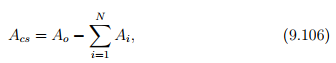where we have assumed that there are N voids. Thus, for the cross section shown in figure 9.22, with the boundary of the cross section in the form of an ellipse centered about origin and oriented such that the major and minor axis coincides with the ex and ey directions  respectively and having two circular shaped voids of radius, r centered at (±xo, 0), the functions f(x, y) and gi(x, y) would be: f(x, y) = x2/a2+y2/b2− 1, g1(x, y) = (x−xo)2+y2−rand g2(x, y) = (x+xo) 2+y2−r2 . Consequently, this body is assumed to occupy a region in Euclidean point space, defined by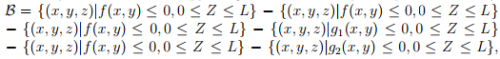where L is a constant. Since, we are interested in the case where in the bar is subjected to pure end torsional moment, the traction boundary conditions are: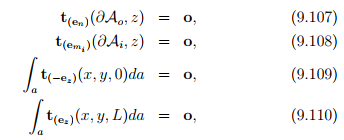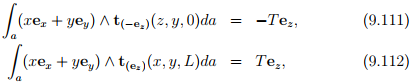where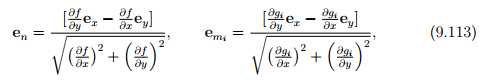and T is a constant. Here en is obtained such that it is in the xy plane, perpendicular to grad(f) and is a unit vector. Similarly, emi is obtained such that it is in the xy plane, perpendicular to grad(gi) and is a unit vector. Recognize that grad(f) gives the tangent vector to the cross section for any point in ∂Aand grad(gi) is the tangent vector to the cross section at any point in ∂Ai . Further, since one end of this body is assumed to be fixed against twisting but free to displace axially and the other end free to displace in all directions, the displacement boundary conditions for this problem is

u(x, y, 0) = uz(x, y)ez,                            (9.114)

where uz is any function of x and y. Here we have assumed that the surface of the body defined by z = 0 is fixed against twisting but free to displace axially and the other surfaces are free to displace in all directions. Thus, we find that the boundary value problem is similar to that in the previous section (section 9.3) except for the additional boundary condition (9.108). Hence, we assume that the stress is as given in equation (9.44), in terms of the Prandtl stress function, φ = φˆ(x, y). Following the standard procedure, as outlined in section 9.3, it can be shown that the stress function should satisfy,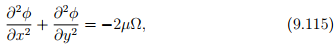where µ is the shear modulus, and Ω is the angle of twist per unit length. For the assumed stress state, (9.44), the boundary condition (9.107) requires that grad(φ) · grad(f) = 0 on ∂Ao. Thus,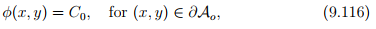where C0 is a constant. As before, since, the stress and displacement fields depend only in the derivative of the stress potential and not its value at a location, it suffices to find this stress function up to a constant. Therefore, we arbitrarily set C0 = 0, understanding that it can take any value and that the stress and displacement field would not change because of this. Hence, equation (9.116) reduces to requiring,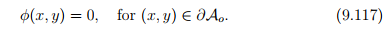The boundary condition (9.108) requires that grad(φ) · grad(gi) = 0 on ∂Ai . Hence,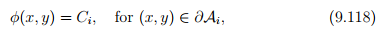where Ci ’s are constants. Though φ is a constant over all free surfaces, the value of this constant can differ between distinct free surfaces, i.e., ∂Ai . Also, recognize that these constants cannot be set to zero, as we have already set C0 = 0. The boundary condition (9.109) and (9.110) for the stress state (9.44) require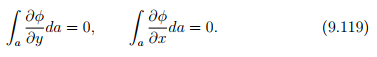Appealing to Green’s theorem for multiply connected domains, (2.278) and (2.279), the above equation (9.119) reduces to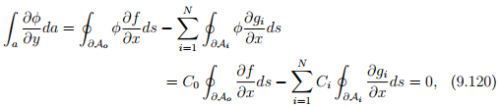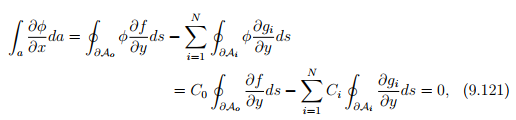where we have used (9.116) and (9.118) and the fact that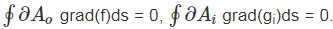Finally, the boundary conditions (9.111) and (9.112) for the stress state (9.44) yields,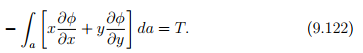Noting that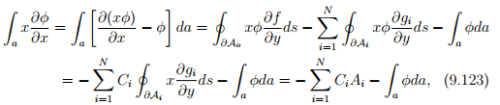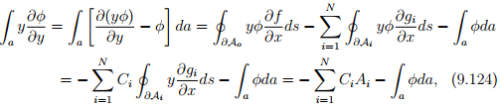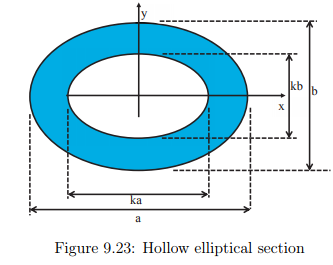where we have used the Green’s theorem for multiply connected domain (2.278) and (2.279) and equations (9.117) and (9.118). Recognize that from Green’s theorem,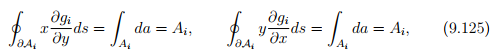where Ai is the area enclosed by the void. Substituting the above equations (9.123) and (9.124) in (9.122) we obtain,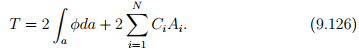Thus, we have to find φ such that the governing equation (9.115) has to hold along with the boundary conditions (9.117) and (9.118). Then, we use equation (9.126) to find the torsional moment required to realize a given angle of twist per unit length, Ω.

Offer running on EduRev: Apply code STAYHOME200 to get INR 200 off on our premium plan EduRev Infinity!

,

,

,

,

,

,

,

,

,

,

,

,

,

,

,

,

,

,

,

,

,

;